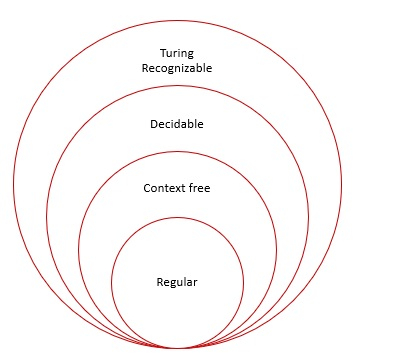# What is Decidability in TOC?

There are two types of languages in the theory of computation (TOC), which are as follows −

• Decidable

• Undecidable

A problem is called decidable, when there is a solution to that problem and also can construct algorithms corresponding to that.

## Example of Decidable Problem

Find all the odd numbers in the range from 1 to 50.

For this problem, we can easily find a solution by constructing an algorithm. In terms of Turing Machine (TM), if a problem is decidable, then the Turing machine halts whether or not it accepts its input.

In terms of finite automata (FA), decidable refers to the problem of testing whether a deterministic finite automata (DFA) accepts an input string. A decidable language corresponds to algorithmically solvable decision problems.

## Decidability Theorem

A language L over Σ is called decidable if,

• There exist a Turing Machine M, that accepts language L

• w€Σ*, M halts

## Decidability

For the Recursive Language

• A language ‘L’ is said to be recursive if there exists a Turing Machine which will accept all the strings in ‘L’ and reject all the strings not in ‘L’.

• The TM will halt every time and give an answer either accepted or rejected for each and every input.

Recursively Enumerable languages −

• A language ‘L’ is said to be recursively enumerable if there exists a TM which accepts and halt for all input in ‘L’.

• But may or may not halt for all input, which are not in ‘L’.

A Language ‘L’ is decidable if it is a recursive language.

All decidable languages are recursive languages and vice versa.

The diagram given below explains the decidable language −